Home Practice
For learners and parents For teachers and schools
Textbooks
Full catalogue
Pricing SupportLog in

We think you are located in United States. Is this correct?

# Rotation transformations

## 24.6 Rotation transformations

A rotation is a transformation that rotates or turns an object. A rotation transformation is a rule that has three components:

• the angle of rotation
• the centre of rotation
• the direction of rotation
angle of rotation
the specific angle through which an object must be turned
centre of rotation
the specific point around which an object must be turned
direction of rotation
the direction in which an object must be turned

For example, we can rotate point $$A$$ by $$90°$$ in a clockwise direction about the origin.

Notice that all three components are included in this transformation statement.

A clockwise direction means turning in the same direction as the hands of a clock. We can also describe clockwise as turning to the right from top to bottom.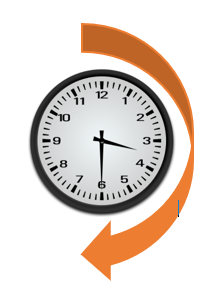An anti-clockwise direction means turning in the opposite direction to the direction of the hands of a clock. We can also describe anti-clockwise as turning to the left from top to bottom.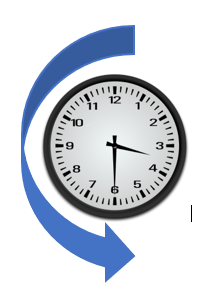### Question 1

In the diagram, $$\triangle MNP$$ is rotated $$180°$$ in a clockwise direction about the origin to produce $$\triangle M'N'P'$$.

1. Write down the coordinates of each vertex of $$\triangle MNP$$ and its image. For example, $$M(0; 0)$$ and $$M'(0; 0)$$.
2. For each vertex of the triangle and its image, what do you notice about the values of the coordinates and their signs?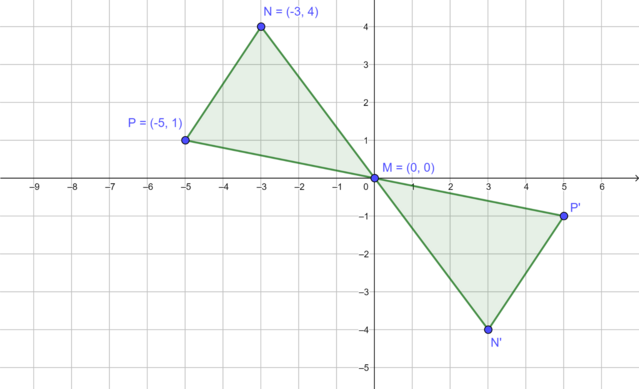### Question 2

In the diagram, $$\triangle TRS$$ is rotated $$90°$$ in an anti-clockwise direction about the origin to produce $$\triangle T'R'S'$$.

1. Write down the coordinates of each vertex of $$\triangle TRS$$ and its image.
2. For each vertex of the triangle and its image, what do you notice about the values of the coordinates and their signs?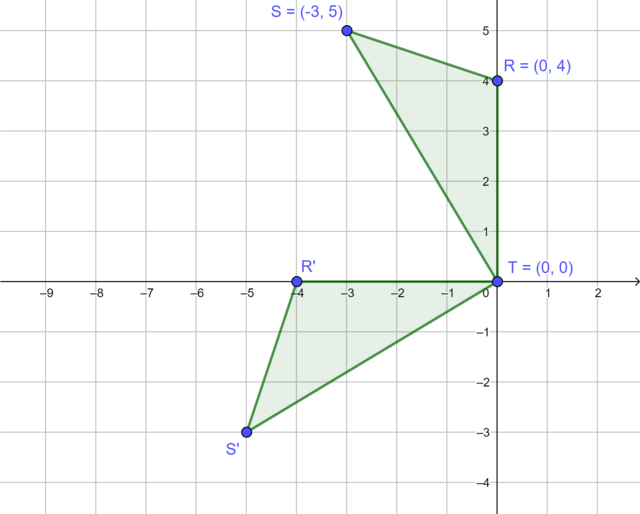### Question 3

In the diagram, $$\triangle JKL$$ is rotated $$90°$$ in a clockwise direction about the origin to produce $$\triangle J'K'L'$$.

1. Write down the coordinates of each vertex of $$\triangle JKL$$ and its image.
2. For each vertex of the triangle and its image, what do you notice about the values of the coordinates and their signs?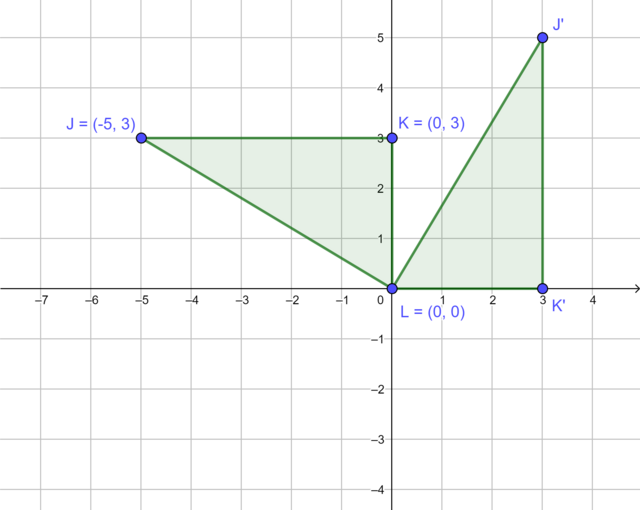When we rotate an object through a certain angle, every point on the object is rotated through the same number of degrees. The diagram shows that $$\triangle X'Y'Z'$$ is the image of $$\triangle XYZ$$ rotated by $$90°$$ in a clockwise direction about the origin. Notice that every vertex of $$\triangle XYZ$$ has been rotated through $$90°$$ to produce the corresponding vertex of $$\triangle X'Y'Z'$$.

Vertex $$X'$$ is the image of vertex $$X$$ rotated by $$90°$$ in a clockwise direction about the origin.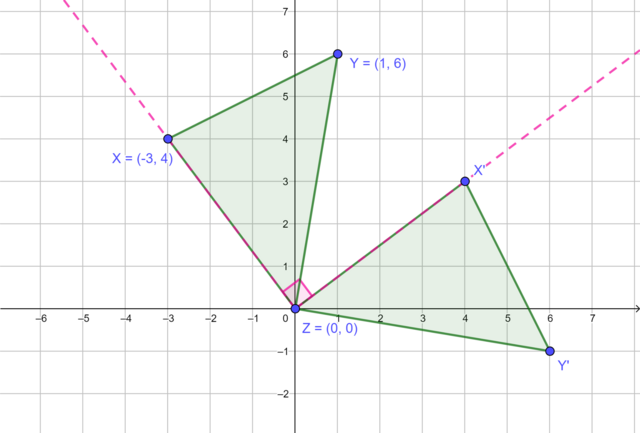Vertex $$Y'$$ is the image of vertex $$Y$$ rotated by $$90°$$ in a clockwise direction about the origin.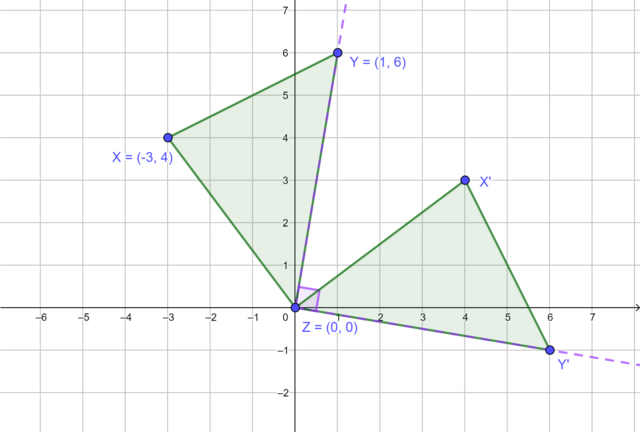Vertex $$Z$$ is on the origin so it stays in the same position.

## Worked example 24.5: Rotating a triangle on the Cartesian plane

1. Plot $$\triangle MNP$$ with vertices $$M(0; 0)$$, $$N(0; 3)$$ and $$P(6; 3)$$ on the Cartesian plane.
2. Draw $$\triangle M'N'P'$$, the image of $$\triangle MNP$$ rotated by $$90°$$ in an anti-clockwise direction about the origin.

### Draw the Cartesian plane and plot the points

First, we look at the values of the coordinates of the vertices of $$\triangle MNP$$ so that we draw the Cartesian plane to an appropriate size. Then, we plot each vertex and draw $$\triangle MNP$$. We notice that vertex $$M$$ is on the origin.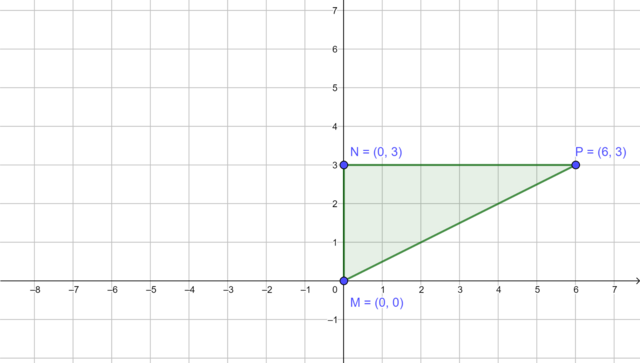### Rotate $$\triangle MNP$$ by $$90°$$ in an anti-clockwise direction about the origin

We rotate each vertex to find its image.

Vertex $$N(0; 3)$$ lies on the $$y$$-axis, so after rotating $$90°$$ in an anti-clockwise direction about the origin, $$N'(-3; 0)$$ lies on the $$x$$-axis.

Vertex $$P(6; 3)$$ lies in the first quadrant. After rotating $$90°$$ in an anti-clockwise direction about the origin, $$P'(-3; 6)$$ lies in the second quadrant.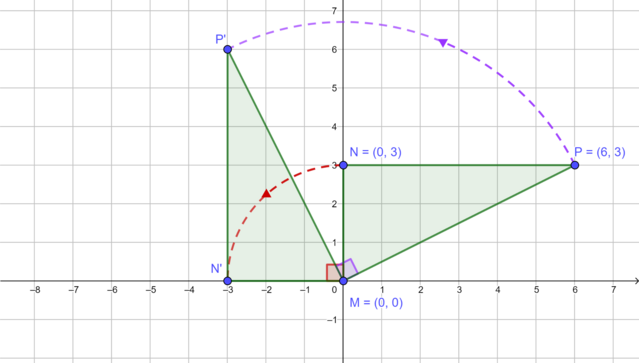# Test yourself now

High marks in science are the key to your success and future plans. Test yourself and learn more on Siyavula Practice.

Exercise 24.2

For each diagram, describe the rotation transformation. Remember to state the angle of rotation, the direction of rotation and the centre of rotation in each case.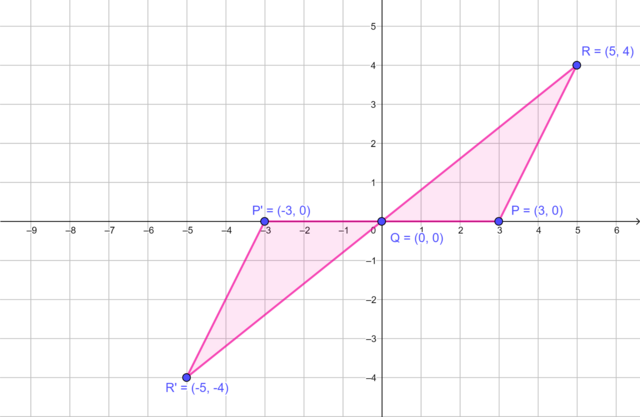$$\triangle P'Q'R'$$, the image of $$\triangle PQR$$ rotated by $$180°$$ in a clockwise (or anti-clockwise) direction about the origin.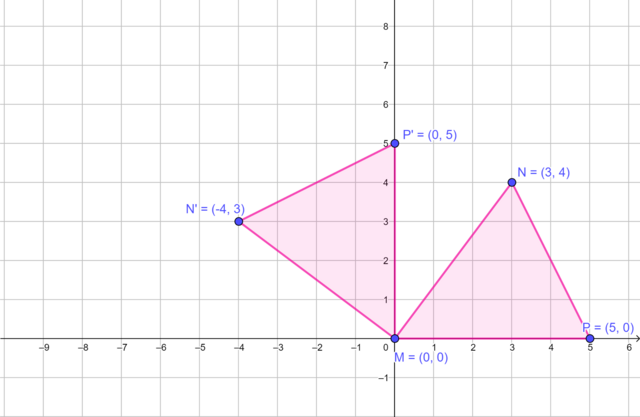$$\triangle M'N'P'$$, the image of $$\triangle MNP$$ rotated by $$90°$$ in an anti-clockwise direction about the origin.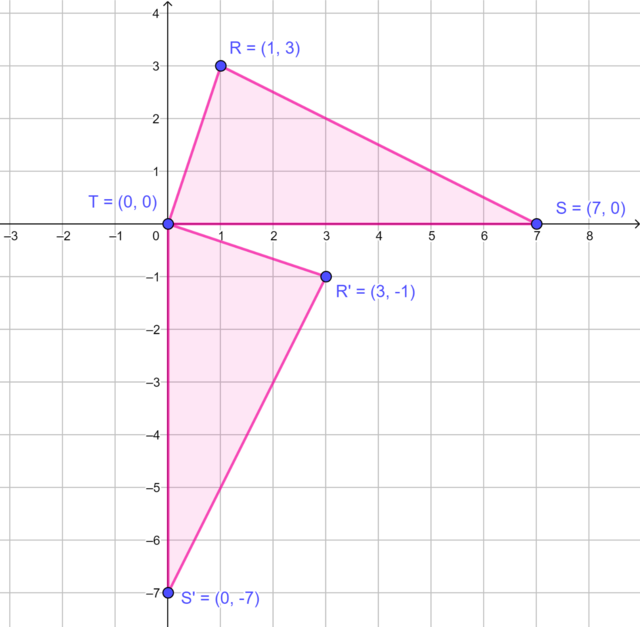$$\triangle R'S'T'$$, the image of $$\triangle RST$$ rotated by $$90°$$ in a clockwise direction about the origin.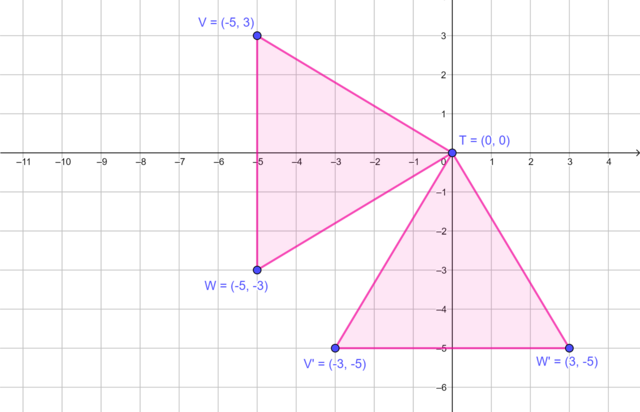$$\triangle T'V'W'$$, the image of $$\triangle TVW$$ rotated by $$90°$$ in an anti-clockwise direction about the origin.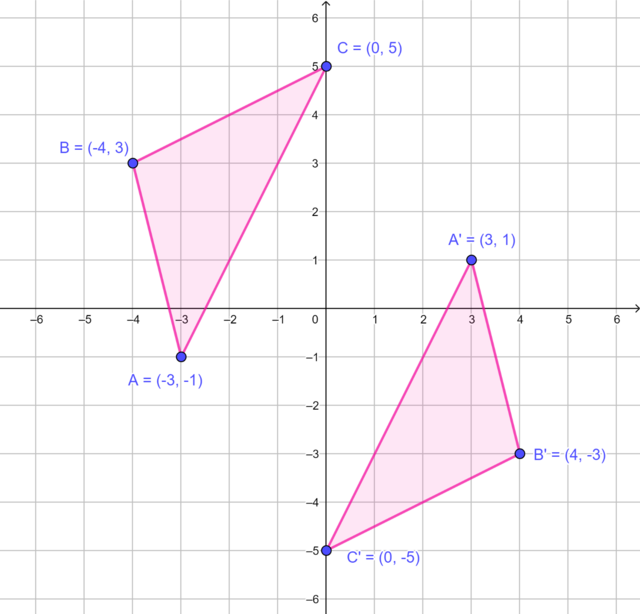$$\triangle A'B'C'$$, the image of $$\triangle ABC$$ rotated by $$180°$$ in a clockwise (or anti-clockwise) direction about the origin.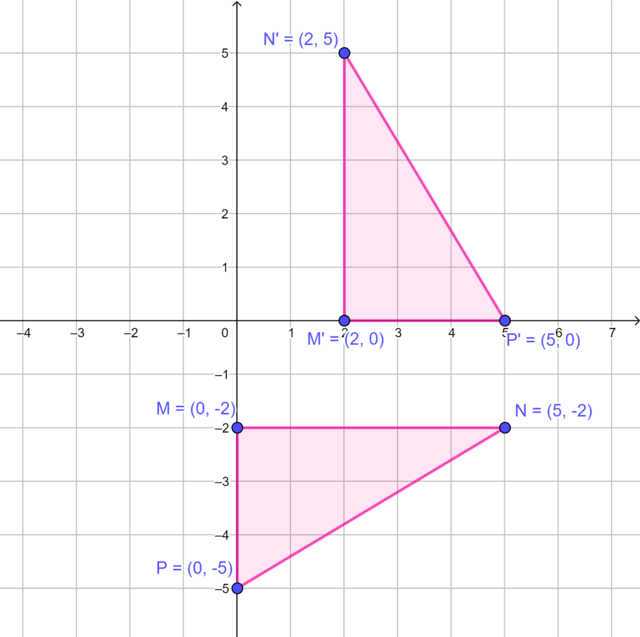$$\triangle M'N'P'$$, the image of $$\triangle MNP$$ rotated by $$90°$$ in an anti-clockwise direction about the origin.

Complete the following on the Cartesian plane:

1. Draw $$\triangle KLM$$ with vertices $$K(-6; 0)$$, $$L(-1; -3)$$ and $$M(0; 0)$$.

2. Draw $$\triangle K'L'M'$$ the image of $$\triangle KLM$$ rotated by $$180°$$ in a clockwise direction about the origin.

1.2.Triangle $$A$$ is the original triangle. Describe the rotation transformation that produces triangle $$B$$ and triangle $$C$$.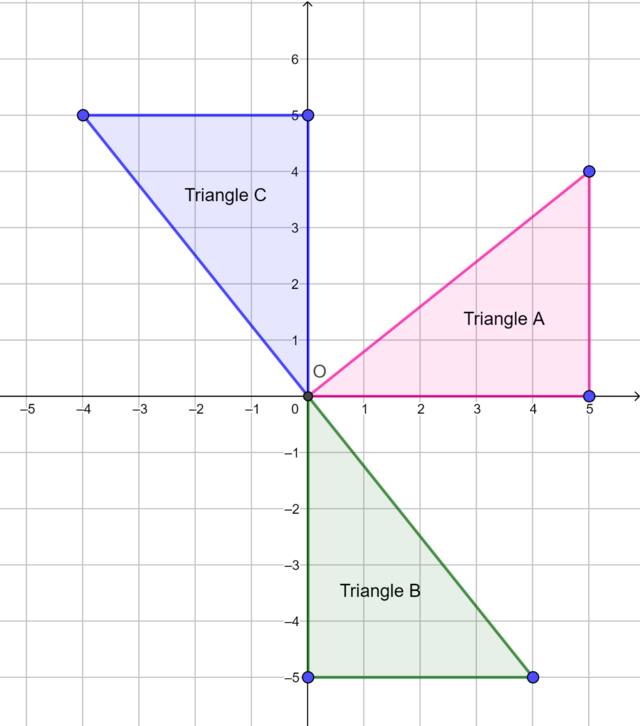1. Triangle $$B$$ is the image of triangle $$A$$ rotated by …

2. Triangle $$C$$ is the image of triangle $$A$$ rotated by …

1. Triangle $$B$$ is the image of triangle $$A$$ rotated by $$90°$$ in a clockwise direction about the origin.

2. Triangle $$C$$ is the image of triangle $$A$$ rotated by $$90°$$ in an anti-clockwise direction about the origin.

Triangle $$A$$ is the original triangle. Describe the rotation transformation that produces triangle $$B$$ and triangle $$C$$.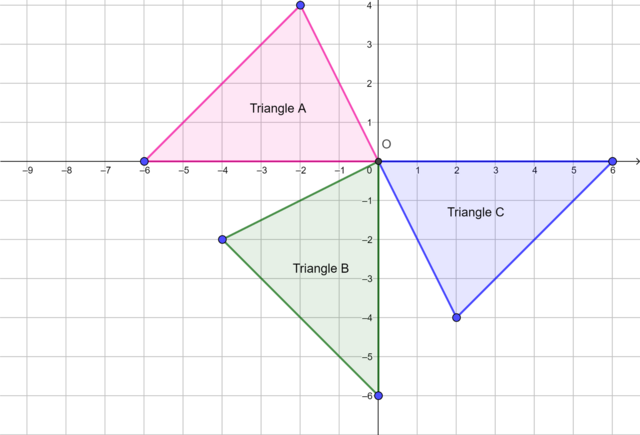1. Triangle $$B$$ is the image of triangle $$A$$ rotated by …

2. Triangle $$C$$ is the image of triangle $$A$$ rotated by …

1. Triangle $$B$$ is the image of triangle $$A$$ rotated by $$90°$$ in an anti-clockwise direction about the origin.

2. Triangle $$C$$ is the image of triangle $$A$$ rotated by $$180°$$ in a clockwise (or anti-clockwise) direction about the origin.

Complete the following on the Cartesian plane:

1. Draw $$\triangle PQR$$ with vertices $$P(5; 0)$$, $$Q(0; 0)$$ and $$R(4; -3)$$.

2. Draw $$\triangle P'Q'R'$$, the image of $$\triangle PQR$$ rotated by $$90°$$ in a clockwise direction about the origin.

1.2.